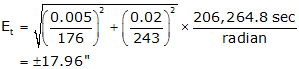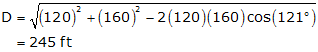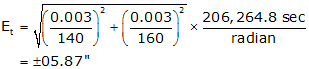## 5. Examples

### a. Example 1

A TSI is used to measure an angle twice direct and reverse with a resulting average angle of 69°35'47". The BS mark is a tripod mounted target with a centering error of ±0.005 ft. The FS mark is a handheld prism pole. The bottom half of the pole is not visible because of the terrain so a centering error of ±0.02 ft will be used. The TSI has a 01" display and its centering error is ±0.003 ft. From the instrument manual, the TSI's stated ISO 17123:3 angle uncertainty is ±06". Approximate horizontal distances to site marks are shown in Figure E-12.Figure E-12 Example 1

What is the expected error in the measured angle?TSI Centering error

To determine this error we need the distance between the targets. This can be computed from the Law of Cosines using the measured angle and distances to the target:thenTarget Centering errorCombinedThe expected error in the angle is ±0°00'19"

Analysis

What's the largest source of error? Target centering, the prism pole in particular, is the largest error contributor.

If we replace the pole with a mark similar to that of the BS, the target centering error drops to ±07.23" and expected angular error to ±00°00'10."

### b. Example 2

The angle shown in Fig 13 will be measured with a TSI having an ISO 17123:3 angle uncertainty of ±05" and centering error of ±0.003 ft. Both BS and FS marks are tripod mounted, also with a centering error of ±0.003 ft.Figure E-13 Example 2

How many D/R sets must be turned to achieve an expected angular error no greater than ±09"?

The only error affected by the number of angles turned is TSI Pointing and Reading, Equation E-3. After substituting TSI Centering and Target Centering errors in Equation E-6, it should be solved for the TSI Pointing and Pointing error. Equation E-3 can then be solved for n.

Compute TSI Centering error, Etsi

Compute distance between targetscompute the errorCompute Target Centering error, EtSet up Equation (E-6) and solve for nSquare both sides, sort terms, and replace Epr with Eqn (E-3)solve for n:6.49 is the minimum value for n to achieve an expected accuracy of ±09"

n is the number of times the angle is measured, direct or reverse. Since we always measure direct and reverse sets, n must be an even integer. Since it's not possible to measure 6.49/2 = 3.245 D/R sets, n must be 6 (3 D/R) or 8 (4 D/R).

For n=6, the angular error, Eang,  is ±09.1", juuuust outside the criterion.
For n=8 the angular error is ±08.8".

To meet the ±09" criteria, measure 4 D/R